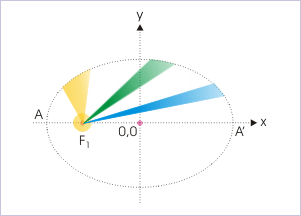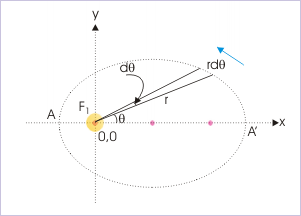# 4.15 Planetary motion  (Page 4/4)

 Page 4 / 4

## Kepler’s laws

Johannes Kepler analyzed Tycho Brahe’s data and proposed three basic laws that govern planetary motion of solar system. The importance of his laws lies in the fact that he gave these laws long before Newton’s laws of motion and gravitation. The brilliance of the Kepler’s laws is remarkable as his laws are consistent with Newton’s laws and conservation laws.

Kepler proposed three laws for planetary motion. First law tells about the nature of orbit. Second law tells about the speed of the planet. Third law tells about time period of revolution.

• Law of orbits
• Law of velocities
• Law of time periods

## Law of orbits

The first law (law of orbits) is stated as :

Law of orbits
The orbit of every planet is an ellipse with the sun at one of the foci.

This law describes the trajectory of a planet, which is an ellipse – not a circle. We have seen that application of mechanics also provides for elliptical trajectory. Only additional thing is that solution of mechanics yields possibilities of other trajectories as well. Thus, we can conclude that Kepler’s law of orbit is consistent with Newtonian mechanics.

We should, however, note that eccentricity of elliptical path is very small for Earth (0.0167) and large for Mercury (0.206) and Pluto (0.25).

## Law of velocities

Law of velocities is a statement of comparative velocities of planets at different positions along the elliptical path.

Law of velocities
The line joining a planet and Sun sweeps equal area in equal times in the planet’s orbit.

This law states that the speed of the planet is not constant as generally might have been conjectured from uniform circular motion. Rather it varies along its path. A given area drawn to the focus is wider when it is closer to Sun. From the figure, it is clear that planet covers smaller arc length when it is away and a larger arc length when it is closer for a given orbital area drawn from the position of Sun. It means that speed of the planet is greater at positions closer to the Sun and smaller at positions away from the Sun.Speed of the planet is greater at positions closer to the Sun and smaller at positions away from the Sun.

Further on close examination, we find that Kepler’s second law, as a matter of fact, is an statement of the conservations of angular momentum. In order to prove this, let us consider a small orbital area as shown in the figure.Time rate of area is statement of conservation of angular momentum.

$\Delta A=\frac{1}{2}X\mathrm{Base}X\mathrm{Height}=\frac{1}{2}r\Delta \theta r=\frac{1}{2}{r}^{2}\Delta \theta$

For infinitesimally small area, the “area speed” of the planet (the time rate at which it sweeps orbital area drawn from the Sun) is :

$⇒\frac{đA}{đt}=\frac{1}{2}{r}^{2}\frac{đ\theta }{đt}=\frac{1}{2}{r}^{2}\omega$

Now, to see the connection of this quantity with angular momentum, let us write the equation of angular momentum :

$L=rX{p}_{\perp }=rm{v}_{\perp }=rmX\omega r=m\omega {r}^{2}$

$⇒\omega {r}^{2}=\frac{L}{m}$

Substituting this expression in the equation of area – speed, we have :

$⇒\frac{đA}{đ\theta }=\frac{L}{2m}$

As no external torque is assumed to exist on the “Sun-planet” system, its angular momentum is conserved. Hence, parameters on the right hand side of the equation i.e. “L” and “m” are constants. This yields that area-speed is constant as proposed by Kepler.

We can interpret the above result other way round also. Kepler’s law says that area-speed of a planet is constant. His observation is based on measured data by Tycho Brahe. It implies that angular momentum of the system remains constant. This means that no external torque applies on the “Sun – planet” system.

## Law of time periods

The law relates time period of the planet with semi major axis of the elliptical trajectory.

Law of time periods
The square of the time period of a planet is proportional to the cube of the semi-major axis.

We have already seen in the case of circular trajectory around a larger mass and also in the case of two body system (see Two body system - circular motion )in which each body is moving along two circular trajectories that time period is given by :

$T=\frac{2\pi {r}^{\frac{3}{2}}}{\sqrt{\left(GM\right)}}$

The expression of time period for elliptical trajectory is similar except that semi-major axis replaces “r”. We have not proved this in the module, but can be so derived. Squaring each of the side and replacing "r" by "a", we have :

$⇒{T}^{2}=\frac{4{\pi }^{2}{a}^{3}}{GM}$

$⇒{T}^{2}\propto {a}^{3}$

## Conclusions

Thus, we conclude the following :

1: The planet follows a elliptical path about Sun.

2: The Sun lies at one of the foci.

3: Gravitational force, centripetal force, linear and angular velocities are variable with the motion.

4: Velocities are maximum at perihelion and minimum at aphelion.

5: Although angular velocity is variable, the angular momentum of the system is conserved.

6: The expression of total mechanical energy is same as in the case of circular motion with the exception that semi major axis, “a”, replaces radius, “r”.

7: The expression of time period is same as in the case of circular motion with the exception that semi major axis, “a”, replaces radius, “r”.

8: Kepler’s three laws are consistent with Newtonian mechanics.

## Acknowldegement

Author wishes to place special thanks to Mr. Mark Prange, Port Isabel, Texas, USA and David F Shively for their valuable editorial suggestions on the subject discussed in this module.

#### Questions & Answers

List the application of projectile
Luther Reply
How can we take advantage of our knowledge about motion?
Kenneth Reply
pls explain what is dimension of 1in length and -1 in time ,what's is there difference between them
Mercy Reply
what are scalars
Abdhool Reply
show that 1w= 10^7ergs^-1
Lawrence Reply
what's lamin's theorems and it's mathematics representative
Yusuf Reply
if the wavelength is double,what is the frequency of the wave
Ekanem Reply
What are the system of units
Jonah Reply
A stone propelled from a catapult with a speed of 50ms-1 attains a height of 100m. Calculate the time of flight, calculate the angle of projection, calculate the range attained
Samson Reply
58asagravitasnal firce
Amar
water boil at 100 and why
isaac Reply
what is upper limit of speed
Riya Reply
what temperature is 0 k
Riya
0k is the lower limit of the themordynamic scale which is equalt to -273 In celcius scale
Mustapha
How MKS system is the subset of SI system?
Clash Reply
which colour has the shortest wavelength in the white light spectrum
Mustapha Reply
how do we add
Jennifer Reply
if x=a-b, a=5.8cm b=3.22 cm find percentage error in x
Abhyanshu Reply
x=5.8-3.22 x=2.58
sajjad

### Read also:

#### Get Jobilize Job Search Mobile App in your pocket Now!

Source:  OpenStax, Physics for k-12. OpenStax CNX. Sep 07, 2009 Download for free at http://cnx.org/content/col10322/1.175
Google Play and the Google Play logo are trademarks of Google Inc.

Notification Switch

Would you like to follow the 'Physics for k-12' conversation and receive update notifications?By OpenStaxBy Michael PittBy Madison ChristianBy Charles JumperBy Angelica LitoBy OpenStaxByBy OpenStaxBy Janet ForresterBy OpenStax